# 快速响应矩阵码（下）

## 纠错码

QR 码采用纠错算法生成一系列纠错码字，添加在数据码字序列之后，使得符号可以在遇到损坏时可以恢复。这就是为什么二维码即使有残缺也可以扫出来。没有残缺创造残缺也要把它扫出来，相信大家见过很多中间带图标的二维码吧。

### 纠错等级

1. 预计的符号质量水平：预计的符号质量等级越低，应用的纠错等级就应越高。
2. 首读率的重要性。
3. 在扫描误读失败后，再次扫描的机会。
4. 印刷符号的空间限制了使用较高的纠错等级。

### 纠错码字的生成

QR 码的纠错使用 Reed–Solomon 编码，有关 Reed–Solomon 码，可以参考这篇文章：http://article.iotxfd.cn/RFID/Reed%20Solomon%20Codes。这里我只大概介绍一下计算过程。

#### 纠错码字的生成多项式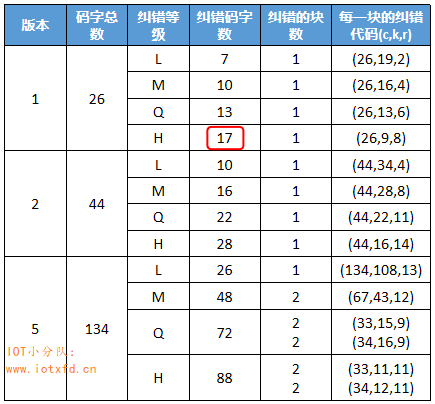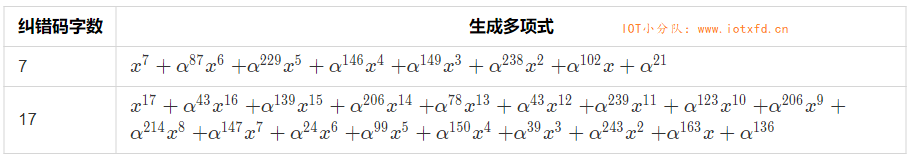#### Reed–Solomon 码的 C# 实现

using System;

namespace QRHelper
{
class ECC
{
const int PRIM = 0x11d;

private static byte[] gfExp = new byte; //逆对数(指数)表
private static byte[] gfLog = new byte; //对数表

static ECC()
{
byte x = 1;
for (int i = 0; i <= 255; i++)
{
gfExp[i] = x;
gfLog[x] = (byte)i;
x = Gf_MultNoLUT(x, 2);
}

for (int i = 255; i < 512; i++)
{
gfExp[i] = gfExp[i - 255];
}
}

//伽罗华域乘法
private static byte Gf_MultNoLUT(int x, int y)
{
int r = 0;
while (y != 0)
{
if ((y & 1) != 0)
{
r ^= x;
}
y >>= 1;
x <<= 1;
if ((x & 256) != 0)
{
x ^= PRIM;
}
}
return (byte)r;
}

//伽罗华域乘法
private static byte GfMul(byte x, byte y)
{
if (x == 0 || y == 0)
{
return 0;
}
return gfExp[gfLog[x] + gfLog[y]];
}

//伽罗华域幂
private static byte GfPow(byte x, int power)
{
return gfExp[(gfLog[x] * power) % 255];
}

//多项式 乘法
private static byte[] GfPolyMul(byte[] p, byte[] q)
{
byte[] r = new byte[p.Length + q.Length - 1];
for (int j = 0; j < q.Length; j++)
{
for (int i = 0; i < p.Length; i++)
{
r[i + j] ^= GfMul(p[i], q[j]);
}
}
return r;
}

/// <summary>
/// 获取纠错码字的生成多项式
/// </summary>
/// <param name="nsym">纠错码字数</param>
/// <returns>由一组数字表示的生成多项式</returns>
public static byte[] RsGeneratorPoly(int nsym)
{
byte[] g = { 1 };
for (int i = 0; i < nsym; i++)
{
g = GfPolyMul(g, new byte[] { 1, GfPow(2, i) });
}
return g;
}

/// <summary>
/// 生成纠错码，并添加在数据码字之后
/// </summary>
/// <param name="msgIn">数据码字</param>
/// <param name="nsym">纠错码字数</param>
/// <returns>数据码字+纠错码字</returns>
public static byte[] RsEncodeMsg(byte[] msgIn, int nsym)
{
if (msgIn.Length + nsym > 255)
{
throw new ArgumentException("数组长度超过 255！");
}
//byte[] gen = generators[(byte)nsym];
byte[] gen = RsGeneratorPoly(nsym);
byte[] msgOut = new byte[msgIn.Length + gen.Length - 1];
Array.Copy(msgIn, 0, msgOut, 0, msgIn.Length);

for (int i = 0; i < msgIn.Length; i++)
{
byte coef = msgOut[i];
if (coef != 0)
{
for (int j = 1; j < gen.Length; j++)
{
msgOut[i + j] ^= GfMul(gen[j], coef);
}
}
}
Array.Copy(msgIn, 0, msgOut, 0, msgIn.Length);

return msgOut;
}
}
}


#### 【例 1 续 2】：生成完整码字

00010000,00100000,00001100,01010110,01100001,10000000,11101100,00010001,11101100

16 进制表示形式为：0x10, 0x20, 0x0C, 0x56, 0x61, 0x80, 0xEC, 0x11, 0xEC

byte[] msgin = { 0x10, 0x20, 0x0C, 0x56, 0x61, 0x80, 0xEC, 0x11, 0xEC };
byte[] msg = ECC.RsEncodeMsg(msgin, 17);


### 构造信息的最终码字序列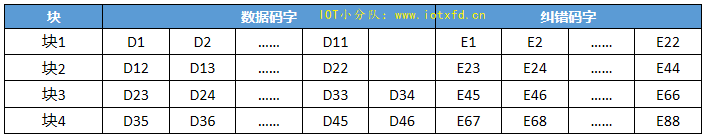D1,D12,D23,D35,D2,D13,D24,D36,...D11,D22,D33,D45,D34,D46,E1,E23,E45,E67,E2,E24,E46,E68,...E22,E44,E66,E88。在某些版本中，需要 3、4 或 7 个剩余位方能填满编码区域模块数，此时需在最后的码字后面加上剩余位（0）。

## 格式信息

### 格式信息的计算

#### BCH 码

BCH 码和 Reed–Solomon 码类似，可以参考 Reed–Solomon 编码这篇文章。Reed–Solomon 码使用多项式除法得出纠错码序列，而 BCH 码就简单得多，它按位运算得出纠错码。BCH(15,5) 表示 BCH 码总长度为 15 位，其中数据码为 5 位，纠错码 10 位。Reed–Solomon 码有生成多项式，BCH 码使用的是生成码：10100110111。使用数据码除以生成码，所得余数就是纠错码。由于 BCH 码的运算很简单，下面演示数据码 00101 的演算过程。

1. 将数据码左移 10 位，凑够 15 位，得到二进制数字：001010000000000
2. 将上面数字除以 10100110111（0x537），使用长除法，如下图所示：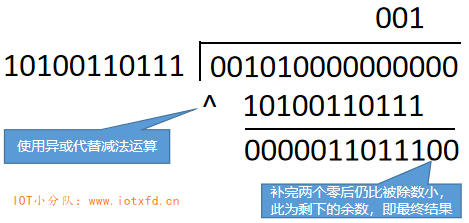1. 将 5 位数据码 00101 与纠错码相连，即得到最终格式信息码：001010011011100（0x14DC）

 //生成 BCH 码
private static int CheckFormat(int fmt)
{
int g = 0x537;
for (int i = 4; i >= 0; i--)
{
if ((fmt & (1 << (i + 10))) != 0)
{
fmt ^= g << i;
}
}
return fmt;
}

/// <summary>
/// 生成 BCH(15,5) 纠错码，并返回完整格式信息码
/// </summary>
/// <param name="data">数据码</param>
/// <returns>返回完整格式信息码</returns>
public static int BCH_15_To_5_Encode(int data)
{
data <<= 10;
return data ^ CheckFormat(data);
}


int code = ECC.BCH_15_To_5_Encode(5);


### 格式信息的绘制

QR 码中有专门的区域绘制格式信息，见下图：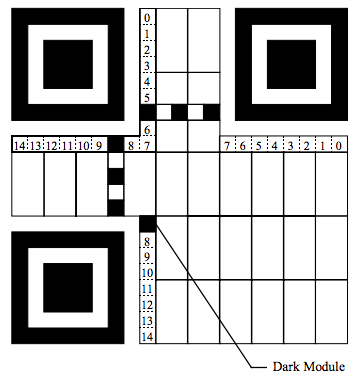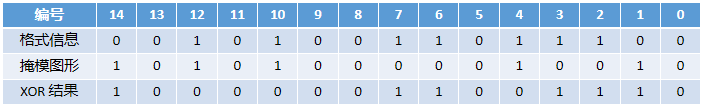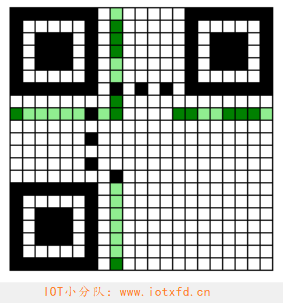#### 【例 1 续 3】：生成格式信息

1. 之前【例 1】中我们选择了纠错等级为 H，查表 5，得到数字：10
2. 假设我们选择的掩模图形参考为 011，则最终数据码为：10011
3. 使用之前的程序将 10011 生成完整格式信息码：100110111000010（0x4DC2）
4. 将生成的格式信息码与 101010000010010（0x5412）进行异或运算，结果为：
001100111010000（0x19D0）
5. 将结果绘制到格式信息区域中，最终结果如下图所示：## 版本信息

### 版本信息的计算

public static int BCH_18_6_Encode(int data)
{
int g = 0x1F25;
int fmt = data << 12;
for (int i = 5; i >= 0; i--)
{
if ((fmt & (1 << (i + 12))) != 0)
{
fmt ^= g << i;
}
}
return (data << 12) ^ fmt;
}


1. 版本号 7 转换为 6 位二进制数据码：000111
2. 将以上数据码左移 12 位，凑够 18 位：000111000000000000
3. 将上面数字除以生成码 1111100100101（0x1F25），得到余数：110010010100
4. 将数据码与得到的余数相连，得到最终版本信息码：000111110010010100

### 版本信息的绘制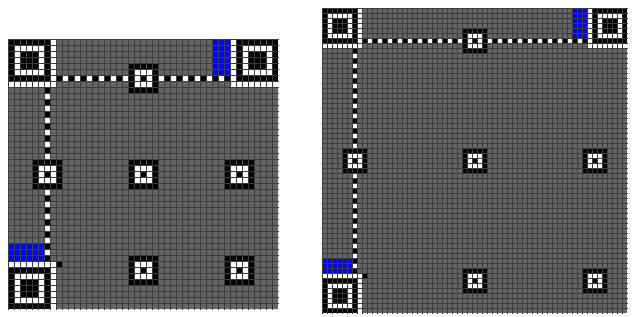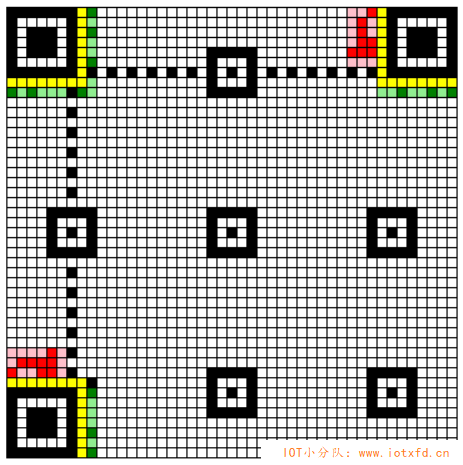## 符号字符的布置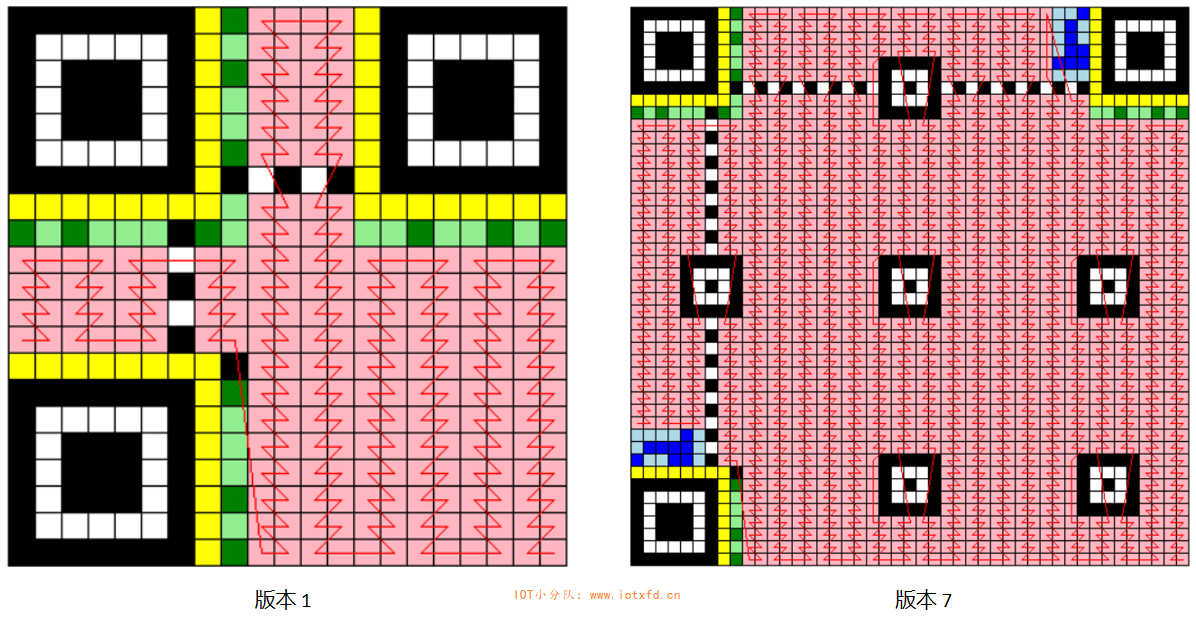### 【例 1 续 4】：布置符号字符

【例 1 续 2】中我们生成了最终的数据码字为：
0x10 0x20 0x0C 0x56 0x61 0x80 0xEC 0x11 0xEC 0x0E 0x9D 0x02 0xC8 0xC2 0x94 0xF3 0xA7 0xAD 0x8D 0xE2 0x0A 0xF4 0xA5 0x2B 0xAC 0xDF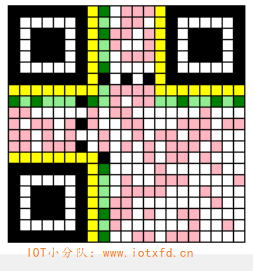## 掩模

QR 码中如果出现大面积的空白或黑块，会导致扫描器识别困难。为了让 QR 图形看起来尽可能凌乱，且尽可能避免位置探测图形中的位图 1011101 的出现，需对 QR 图形进行掩模操作，步骤如下：

1. 掩模不用于功能图形及格式图形：寻像图形、定位图形、校正图形、位置探测图形分隔符、格式信息和版本信息。
2. 数据码字与掩模图形进行 XOR 操作后再进行绘制。
3. 对每个结果图形的不合要求的部分记分，以评估这些结果。
4. 选择得分最低的图形。

### 掩模图形

000 (i + j) mod 2 = 0
001 i mod 2 = 0
010 j mod 3 = 0
011 (i + j) mod 3 = 0
100 ((i/2)+(j/3)) mod 2 = 0
101 (i × j) mod 2 + (i × j) mod 3 = 0
110 ((i × j) mod 2 + (i × j) mod 3) mod 2 = 0
111 ((i × j) mod 3 + (i + j) mod 2) mod 2 = 0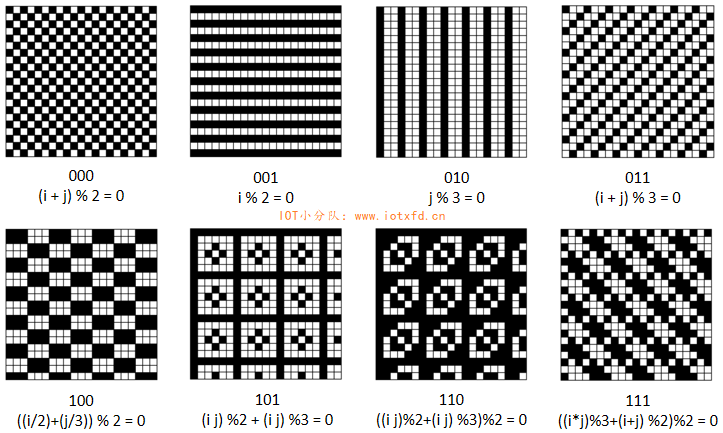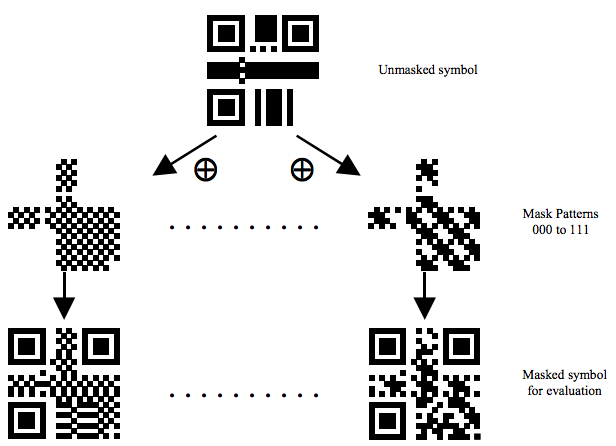### 【例 1 续 5】：加入掩模图形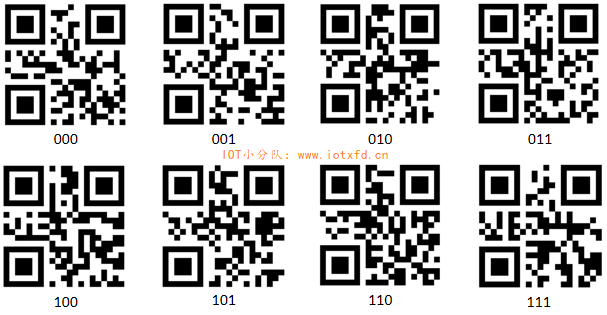posted @ 2019-10-16 21:21  abatei  阅读(5841)  评论(0编辑  收藏  举报